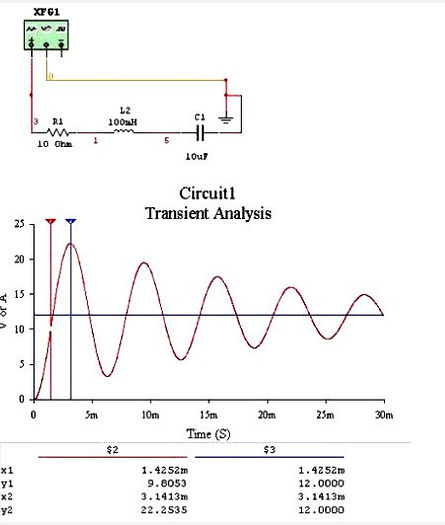naver-site-verification: naver47238a1b6bfbb19a2fd4b619734fa9a6.html
top of page

# Transients in RLC-circuits calculated using the Ti-84 Plus

In RLC electric circuits, special phenomena can occur (such as resonance). The behavior of the RLC circuit depends on the values of R, L and C. With the second order differential solver program and the post process program the phenomena can be investigated which is important for electrical engineering.

For extra information about the second order differential equation solver, go to :

### Example (see scheme)  with: R1=10 Ω, L1=100 mH, C1=10 uF, V1=12V DC### Formulas  for the electric circuit and applied in the Ti-Basic programFor this case, it yields :

y=Uc1,   A=L1C1=1E-6,   B-=R1C1=1E-4,

C=1,     f(x) =12V

For numerical processes, the step size is an important parameter.  For this example, next step size is chosen.

Step size dx < √(A/C) /100 = √((1E-6))/100 =0.001/100=1E-5 sec or dx <(B/C)/20 = 0.025 sec

Take the smallest step size of dx which is dx=0.00001=1E-5 sec for this example.

The result obtained with the Ti show that at 3.12 msec the maximum capacitor voltage equals 22.25 V. The Ti results approach the results of Multisim and the analytical solution extremely well. At t=0.22 sec, values of the numerical program and the analytical solution agree.## Results Multisim                                                          analytical solutionNews July 2023: The third version DV2ETRV3 is now available with 999 calculated points stored.